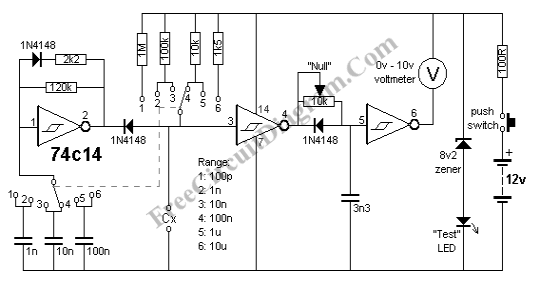# Capacitance MeterCapacitance meter can be very helpful in identifying old components where its mark has been erased or became unreadable. Such instrument can be quite expensive, but fortunately we can build it easily with much lower cost. The capacitance meter described here can measure any capacitor between 1pF and 10uF. Here is the schematic diagram of the circuit:You can just use a connector  for the voltmeter, and the circuit becomes a capacitance meter adapter for your general purpose voltmeter. Just plug the input of your voltmeter to this capacitance meter circuit and now your voltmeter becomes capacitance meter.  You can use an analog voltmeter or digital voltmeter. If you use a digital voltmeter, make sure your digital voltmeter is a dual/single  slope type reading, since it has an inherent averaging function. If your digital voltmeter is a fast sampling type, then you need to insert a resitor-capacitor filter between this capacitance meter circuit and the voltmeter. A 1k resitor and a 10uF electrolytic capacitor sould be enough for the filter. Analog voltmeter doesn’t need any filter since its mechanical inertia acts like a low-pass filter in nature. More information can be found at [Source: talkingelectronics.com]